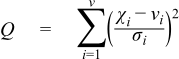#Boost C++ Libraries

...one of the most highly regarded and expertly designed C++ library projects in the world.

This is the documentation for an old version of Boost. Click here to view this page for the latest version.

#### Chi Squared Distribution

`#include <boost/math/distributions/chi_squared.hpp>`
```namespace boost{ namespace math{

template <class RealType = double,
class Policy   = policies::policy<> >
class chi_squared_distribution;

typedef chi_squared_distribution<> chi_squared;

template <class RealType, class Policy>
class chi_squared_distribution
{
public:
typedef RealType  value_type;
typedef Policy    policy_type;

// Constructor:
chi_squared_distribution(RealType i);

// Accessor to parameter:
RealType degrees_of_freedom()const;

// Parameter estimation:
static RealType find_degrees_of_freedom(
RealType difference_from_mean,
RealType alpha,
RealType beta,
RealType sd,
RealType hint = 100);
};

}} // namespaces
```

The Chi-Squared distribution is one of the most widely used distributions in statistical tests. If χi   are ν   independent, normally distributed random variables with means μi   and variances σi2, then the random variable:is distributed according to the Chi-Squared distribution.

The Chi-Squared distribution is a special case of the gamma distribution and has a single parameter ν   that specifies the number of degrees of freedom. The following graph illustrates how the distribution changes for different values of ν:##### Member Functions
```chi_squared_distribution(RealType v);
```

Constructs a Chi-Squared distribution with v degrees of freedom.

Requires v > 0, otherwise calls domain_error.

```RealType degrees_of_freedom()const;
```

Returns the parameter v from which this object was constructed.

```static RealType find_degrees_of_freedom(
RealType difference_from_variance,
RealType alpha,
RealType beta,
RealType variance,
RealType hint = 100);
```

Estimates the sample size required to detect a difference from a nominal variance in a Chi-Squared test for equal standard deviations.

difference_from_variance

The difference from the assumed nominal variance that is to be detected: Note that the sign of this value is critical, see below.

alpha

The maximum acceptable risk of rejecting the null hypothesis when it is in fact true.

beta

The maximum acceptable risk of falsely failing to reject the null hypothesis.

variance

The nominal variance being tested against.

hint

An optional hint on where to start looking for a result: the current sample size would be a good choice.

Note that this calculation works with variances and not standard deviations.

The sign of the parameter difference_from_variance is important: the Chi Squared distribution is asymmetric, and the caller must decide in advance whether they are testing for a variance greater than a nominal value (positive difference_from_variance) or testing for a variance less than a nominal value (negative difference_from_variance). If the latter, then obviously it is a requirement that ```variance + difference_from_variance > 0```, since no sample can have a negative variance!

This procedure uses the method in Diamond, W. J. (1989). Practical Experiment Designs, Van-Nostrand Reinhold, New York.

See also section on Sample sizes required in the NIST Engineering Statistics Handbook, Section 7.2.3.2.

##### Non-member Accessors

All the usual non-member accessor functions that are generic to all distributions are supported: Cumulative Distribution Function, Probability Density Function, Quantile, Hazard Function, Cumulative Hazard Function, mean, median, mode, variance, standard deviation, skewness, kurtosis, kurtosis_excess, range and support.

(We have followed the usual restriction of the mode to degrees of freedom >= 2, but note that the maximum of the pdf is actually zero for degrees of freedom from 2 down to 0, and provide an extended definition that would avoid a discontinuity in the mode as alternative code in a comment).

The domain of the random variable is [0, +∞].

##### Examples

Various worked examples are available illustrating the use of the Chi Squared Distribution.

##### Accuracy

The Chi-Squared distribution is implemented in terms of the incomplete gamma functions: please refer to the accuracy data for those functions.

##### Implementation

In the following table v is the number of degrees of freedom of the distribution, x is the random variate, p is the probability, and q = 1-p.

Function

Implementation Notes

pdf

Using the relation: pdf = gamma_p_derivative(v / 2, x / 2) / 2

cdf

Using the relation: p = gamma_p(v / 2, x / 2)

cdf complement

Using the relation: q = gamma_q(v / 2, x / 2)

quantile

Using the relation: x = 2 * gamma_p_inv(v / 2, p)

quantile from the complement

Using the relation: x = 2 * gamma_q_inv(v / 2, p)

mean

v

variance

2v

mode

v - 2 (if v >= 2)

skewness

2 * sqrt(2 / v) == sqrt(8 / v)

kurtosis

3 + 12 / v

kurtosis excess

12 / v

##### References
 Copyright © 2006-2010, 2012, 2013 Paul A. Bristow, Christopher Kormanyos, Hubert Holin, Bruno Lalande, John Maddock, Johan Råde, Gautam Sewani, Benjamin Sobotta, Thijs van den Berg, Daryle Walker and Xiaogang Zhang Distributed under the Boost Software License, Version 1.0. (See accompanying file LICENSE_1_0.txt or copy at http://www.boost.org/LICENSE_1_0.txt)